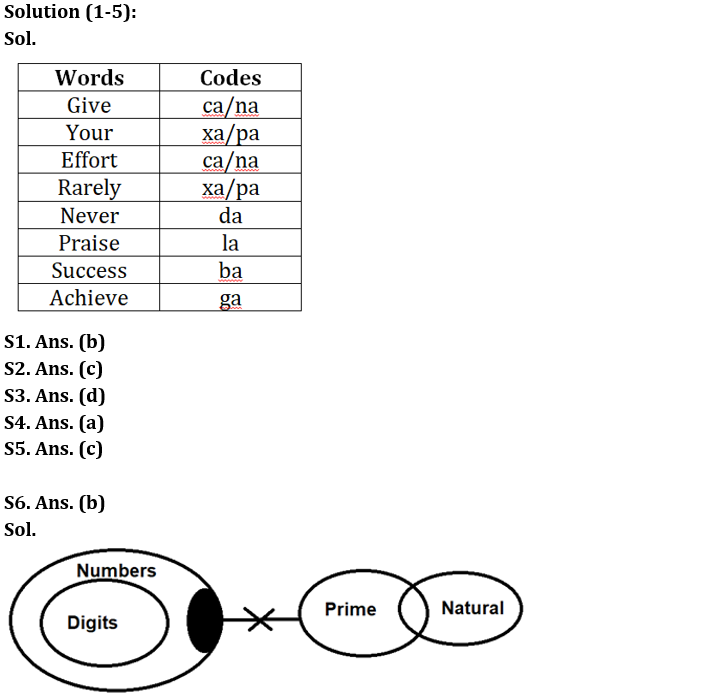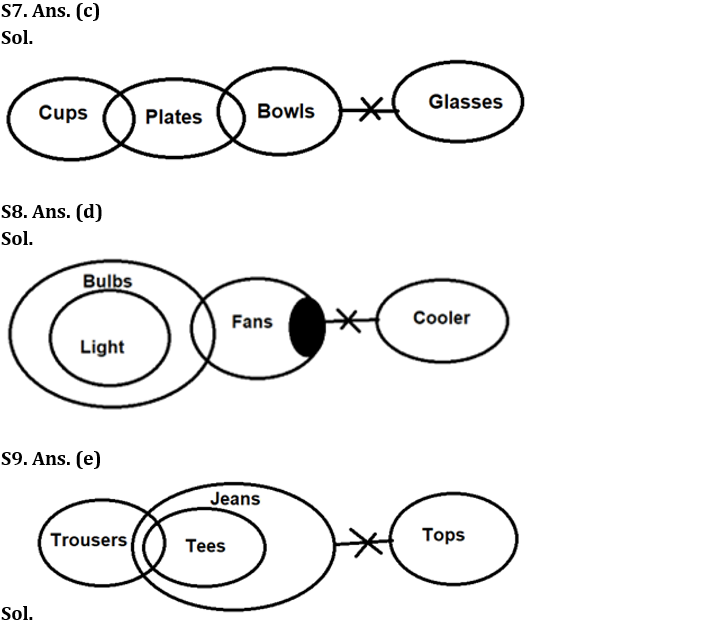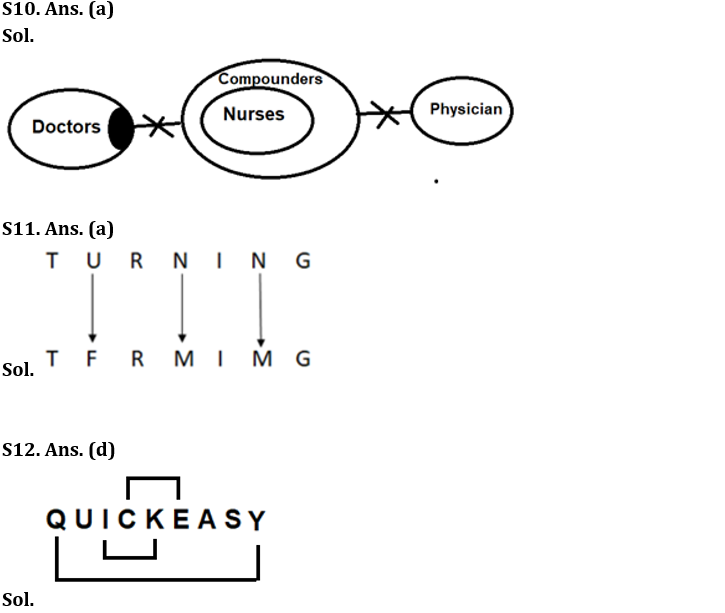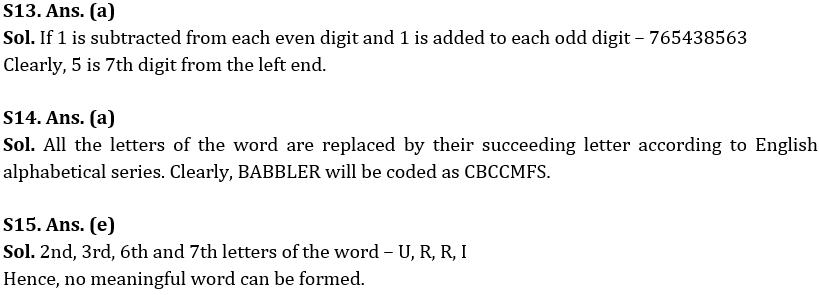Latest Banking jobs   »

# Reasoning Ability Quiz For IBPS RRB PO Prelims 2022- 23rd June

Directions (1-5): Study the following information carefully and answer the questions accordingly.
In a certain code language,
“Give your efforts rarely” is coded as “xa ca na pa”
“Never your praise rarely” is coded as “da la pa xa”
“Success give efforts never” is coded as “ca ba na da”
“Achieve your praise rarely” is coded as “ga xa pa la”

Q1. What is the code of “never”?
(a) la
(b) da
(c) ca
(d) na
(e) None of these

Q2. What could be the code of “achieve give”?
(a) ba ca
(b) da na
(c) ca ga
(d) xa pa
(e) None of these

Q3. What is the code of “rarely your success”?
(a) ba xa ca
(b) xa pa ga
(c) xa ba ca
(d) xa pa ba
(e) None of these

Q4. If the code of “efforts never” is “na da”, then what will be the code of “give praise”?
(a) ca la
(b) la ba
(c) ga ba
(d) ca na
(e) None of these

Q5. What is the code of “your”?
(a) xa
(b) la
(c) Cannot be determined
(d) ga
(e) None of these

Directions (6-10): In the question below three statements are given followed by the conclusions. You have to take the given statements to be true even if they seem to be at variance with commonly known facts. Read all the conclusions and then decide which of the given conclusions logically follow from the given statements disregarding commonly known facts.

Q6. Statements: All digits are numbers. Some numbers are not prime. Only a few prime are natural.
Conclusions: I. Some digits are not prime
II. Some prime are not natural
(a) Only conclusion I follows
(b) Only conclusion II follows
(c) Either conclusion I or II follows
(d) Both conclusions I and II follow
(e) Neither conclusion I nor II follows

Q7. Statements: Some Cups are Plates. Only a few Plates are Bowls. No Bowls are Glasses.
Conclusions: I. Some Plates are Glasses
II. No Plates are Glasses
(a) Only conclusion I follows
(b) Only conclusion II follows
(c) Either conclusion I or II follows
(d) Both conclusions I and II follow
(e) Neither conclusion I nor II follows

Q8. Statements: All Light is Bulb. Only a few Bulbs are Fans. Some Fans are not Coolers.
Conclusions: I. Some Lights can be Fans
II. Some bulb is not Fan
(a) Only conclusion I follows
(b) Only conclusion II follows
(c) Either conclusion I or II follows
(d) Both conclusions I and II follow
(e) Neither conclusion I nor II follows

Q9. Statements: Only a few Trousers are Tees. All Tees are Jeans. No Jeans are Tops.
Conclusions: I. All Trousers are Jeans
II. Some Tees can be Tops
(a) Only conclusion I follows
(b) Only conclusion II follows
(c) Either conclusion I or II follows
(d) Both conclusions I and II follow
(e) Neither conclusion I nor II follows

Q10. Statements: Some Doctors are not Compounders. All Nurses are Compounders. No Compounder is Physician.
Conclusions: I. No Nurses are Physician
II. Some Compounder is not Doctor
(a) Only conclusion I follows
(b) Only conclusion II follows
(c) Either conclusion I or II follows
(d) Both conclusions I and II follow
(e) Neither conclusion I nor II follows

Q11. In a certain code language, if ‘PUNCTURE’ is coded as ‘PFNXTFRV’ and ‘FLAMES’ is coded as ‘FOANEH’, then what is the code for ‘TURNING’?
(a) TFRMIMG
(b) TFRMIGM
(c) TFRIMMG
(d) TFMRIMG
(e) None of these

Q12. How many such pairs of letters are there in the word ‘QUICKEASY’ each of which has as many letters between them in the word as in the English alphabet (Both forward and backward)?
(a) None
(b) One
(c) Two
(d) Three
(e) More than three

Q13. If in the number ‘856347654’, 1 is subtracted from each even digit and 1 is added to each odd digit, then which digit is 7th from the left end?
(a) 5
(b) 8
(c) 4
(d) 3
(e) None of these

Q14. If in a certain way ABANDON is coded as BCBOEPO, then how BABBLER will be coded in the same manner as?
(a) CBCCMFS
(b) CCBCFMS
(c) CFMSCCB
(d) FMSCBCC
(e) None of these

Q15. If it is possible to make a meaningful word, using the 2nd, 3rd, 6th and 7th letters of the word “TURMERIC’, then what is the second letter of the meaningful word? If no such meaningful word be formed, mark the answer as ‘Z’. If more than one meaningful word can be formed mark the answer as ‘K’.
(a) R
(b) I
(c) U
(d) K
(e) Z

Solutions#### Congratulations!